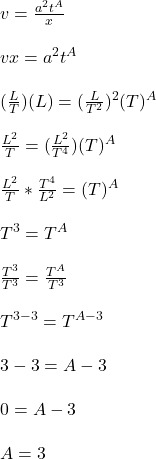## Consider position [x] = L, time [t] = T, velocity [v] = L/T and acceleration [a] = L/T 2 . Find the exponent A in the equation v = a^2 t^ A

Question

Consider position [x] = L, time [t] = T, velocity [v] = L/T and acceleration [a] = L/T 2 . Find the exponent A in the equation v = a^2 t^ A /x

in progress 0
6 months 2021-08-02T07:59:51+00:00 1 Answers 30 views 0

## Answers ( )